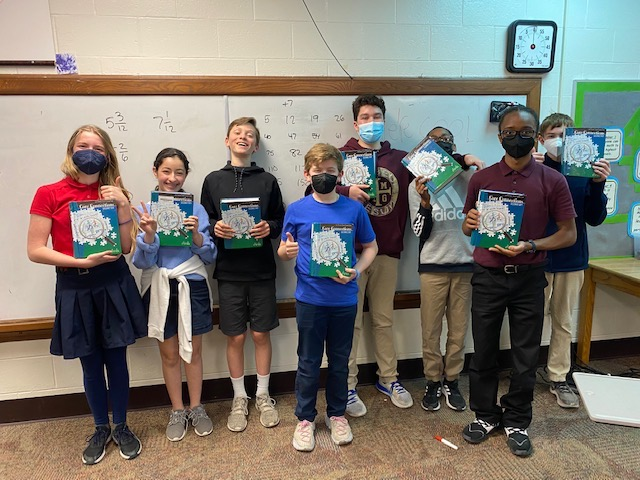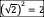# Geometry – Unit Polygons (part one)

Abstract: In this series of posts, we will explore areas of polygons with a radius of one. We will begin by showing that the areas will converge on π and perimeters will converge on 2π. In upcoming parts, we will explore questions that came up in my geometry class such as: Is there a relevant pattern in these areas? If we graphed the areas and perimeters, what would it reveal? How do we interpret a regular polygon with 4.5 sides? Each of these and others will be examined in later parts.

My favorite (and most productive) classes usually start with an activity that goes off on a tangent at the insistence of my students.  It happens most often in a class where they have been project-based since day one.  Here is an example from my Geometry class.

Our activity for the day was to find the areas and perimeters of regular polygons of radius one from 3 sides to 150 sides.  This was an activity in the CPM Geometry Book.  I will call these shapes unit polygons.  If you know of a better name or have seen this term used somewhere, please let me know.  Of course, the objective of this activity is to discover that the area will converge on π and the perimeter will converge on 2π since a unit polygon with infinite sides is a circle.

I find that the best way to do this activity is to withhold the objective, so you don’t spoil the surprise.  This is one area we might find ourselves in conflict with our administrators.  Math can be surprising.  That is part of the fun and a powerful way to engage a concept.  It is worth losing a point or two on your evaluation for not “clearly defining the objectives”.  Encourage your administrator to stay for the whole class and maybe even join one of the groups.  They will get it.  (Remind them that math learning is not age dependent.)

I had four groups, so I assigned two unit polygons to each group.  I put a table on the board and made sure I didn’t assign consecutive numbers so we could discover the pattern as a class.

Almost Immediately, they recognized that the group with the unit square had the easiest one.  A quick review of isosceles right triangles gives an area of.

They also recognized that the unit hexagon was easy to solve with equilateral and 30-60-90 triangles.

Some quick Vocabulary:

Circumradius – The radius of a circumscribed circle.  Also, the line segment that connects the center to a vertex.

Apothem – The radius of an inscribed circle.  Also, the line segment that connects the center perpendicular to the opposite side.  In the triangle, it is an altitude.

Pocket Question to get them thinking:  What do you have when the Apothem is equal to the Circumradius?  A Circle.  I know it sounds trivial, but it will get them to stop and think a second. When they get this, it makes them feel like they are on track. I love trivial pocket questions! Especially when I am circulating through the groups. I meet them at their eye level, ask the question and wait for an answer. The answer may surprise you.

Side Thought:  A few students might deduce that the Area of the Unit Hexagon is six times the Area of the Unit Equilateral Triangle because it is made up of six equilateral triangles.  This is not the case because it will have a different circumradius as follows:

You can see that the hexagon is made up of six much smaller equilateral triangles. You should keep this conversation visual or ask them to calculate each triangle area. Or both.

Here is the unit heptagon.  (I always tell my students “if you can’t remember the name of the polygon, it is a Heptagon”).  it only has one more side than the hexagon, but it is more challenging since it has an odd number of sides.

Different groups used different methods as follows:

• Some used the half angle and a little bit of right triangle trigonometry to find the area and perimeter as follows:

The perimeter is easy to calculate using the base above times 14.

• Some used the central and the trig formula
• Some used the law of cosines to find the base of the triangle and found the perimeter as follows:

Then I called all the groups attention to the board in the front of g until they see it themselves. Usually, either another group says something or the group interrupts me with the corrected value.

After we have collected up all the areas, ask if they see a pattern. They usually see it converging on π. And ask the trivial question: “What do you call a regular polygon with an infinite number of sides.” Look for an infrequent hand. It will be there. I promise.

Now ask them to think about the perimeters. What will it converge on? Have them discuss it in groups for one minute. Watch the time. At the end of the minute, you will have a few ideas.

Start collecting perimeters and filling in the table. Stop after eight sides and give them another minute if they need it. If no group gets 2π, ask them what we call the perimeter of a circle? (circumference). Then, how do you find the circumference of a circle? Eventually, they will land on 2π.

The entire table on the board looked like this:

This brings us to our first question.  (or “Notice/Wonder” if you prefer).

π is a significant number in mathematics.  What about those other areas?  Such as 2.598 for a hexagon.  Are they analogous to π for a circle?  Could we say π6=2.598?  Is there a real-world or visual representation of this?  (OK that was several questions).

These were the questions in my class.  A beautiful tangent.   Well worth giving up one class or even a week to pursue.

If you can get to this point in one day, you are doing well.  Try to time it so they will leave with the questions in their heads.  (Tell them to go to bed early.

Next week, we will look at what happened with these questions and maybe even generate more questions.  Inquiry-based learning.  Socratic Math.  I can’t wait!

••